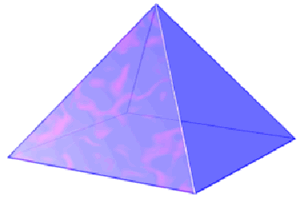# 正四棱锥

• 有 5面
• 4个侧面是三角形
• 底面是正方形
• 有 5个顶点（角）
• 有 8条棱

## 表面积### 例子：正四棱锥的侧面相同，斜高是 3，底面是边长为 5 的正方形

 表面积 = [底面积] + 1 2 × 周长 × [斜高] = [5 × 5] + 1 2 × (5+5+5+5) × 3 = 25 + 1 2 × 20 × 3 = 25 + 30 = 55

## 体积

### 例子：底面是边长为 5 的正方形，高是 6

 体积 = 1 3 × [底面积] × 高 = 1 3 × [5 × 5] × 6 = 1 3 × 150 = 50